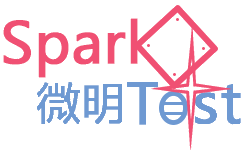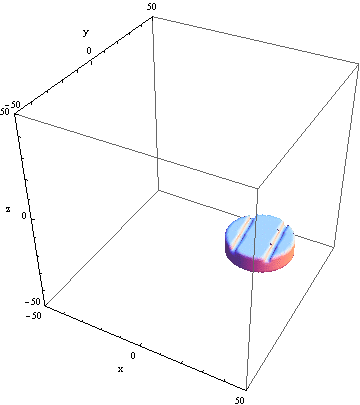微明火花试验网   WeiMing Intrinsic Safety Spark Test

\[Alpha] = 0.0011 h1 = 6; r = 15; h2 = 8;r1 = 2.5; l1 = 60;L1 = 31;L2 = \ 10; \[Theta] = 0.999 ;a = Pi/4(*常数*);\[Theta]1 = ArcCos[6.5/r]; \[Theta]2 = ArcCos[8.5/r]; \[Alpha] = Mod[\[Alpha], 2 Pi]; If[Pi < \[Alpha] < 3 Pi/2, \[Alpha] = \[Alpha] - Pi]; If[3 Pi/2 < \[Alpha] < 2 Pi, \[Alpha] = \[Alpha] - Pi]; xg01 = r Cos[\[Alpha] + \[Theta]1]; yg01 = r Sin[\[Alpha] + \[Theta]1]; xg02 = r Cos[\[Alpha] + \[Theta]2]; yg02 = r Sin[\[Alpha] + \[Theta]2]; xg11 = r Cos[\[Alpha] + Pi - \[Theta]1]; yg11 = r Sin[\[Alpha] + Pi - \[Theta]1]; xg12 = r Cos[\[Alpha] + Pi - \[Theta]2]; yg12 = r Sin[\[Alpha] + Pi - \[Theta]2]; sg1[\[Alpha]0_] = If[(0 < \[Alpha]0 < Pi/2) || (Pi/2 < \[Alpha]0 < Pi), -1/ Tan[\[Alpha]0]]; sg = sg1[\[Alpha]] pp = 100;box = 50; If[\[Alpha] == 0 || \[Alpha] == Pi, Print["0,Pi"]; p1 = RegionPlot3D[(x - L1)^2 + y^2 <= r^2 && -r <= y <= r && Not[(6.5 + L1 <= x <= 8.5 + L1 || -8.5 + L1 <= x <= -6.5 + L1) && -(h1/2 + L2 + 2) <= z <= -(h1/2 + L2)] && -(h1/2 + L2 + h2) <= z <= -(h1/2 + L2), {x, -box, box}, {y, -box, box}, {z, -box, box}, Mesh -> None, PlotPoints -> pp, AxesLabel -> {"x", "y", "z"}];, If[\[Alpha] == Pi/2 || \[Alpha] == 3 Pi/2, Print["Pi/2"]; p1 = RegionPlot3D[(x - L1)^2 + y^2 <= r^2 && -r <= y <= r && Not[(6.5 <= y <= 8.5 || -8.5 <= y <= -6.5) && -(h1/2 + L2 + 2) <= z <= -(h1/2 + L2)] && -(h1/2 + L2 + h2) <= z <= -(h1/2 + L2), {x, -box, box}, {y, -box, box}, {z, -box, box}, Mesh -> None, PlotPoints -> pp, AxesLabel -> {"x", "y", "z"}, WorkingPrecision -> 60];, Print["other"]; p1 = RegionPlot3D[(x - L1)^2 + y^2 <= r^2 && -r <= y <= r && Not[sg (x - L1) + yg01 - sg xg01 <= y <= sg (x - L1) + yg02 - sg xg02 && -(h1/2 + L2 + 2) <= z <= -(h1/2 + L2)] && Not[sg (x - L1) + yg12 - sg xg12 <= y <= sg (x - L1) + yg11 - sg xg11 && -(h1/2 + L2 + 2) <= z <= -(h1/2 + L2)] && -(h1/2 + L2 + h2) <= z <= -(h1/2 + L2), {x, -box, box}, {y, -box, box}, {z, -box, box}, Mesh -> None, PlotPoints -> pp, PlotRange -> box, AxesLabel -> {"x", "y", "z"}]]]; Show[p1]

Mathematica生成的3D齿轮